$$\newcommand{\id}{\mathrm{id}}$$ $$\newcommand{\Span}{\mathrm{span}}$$ $$\newcommand{\kernel}{\mathrm{null}\,}$$ $$\newcommand{\range}{\mathrm{range}\,}$$ $$\newcommand{\RealPart}{\mathrm{Re}}$$ $$\newcommand{\ImaginaryPart}{\mathrm{Im}}$$ $$\newcommand{\Argument}{\mathrm{Arg}}$$ $$\newcommand{\norm}{\| #1 \|}$$ $$\newcommand{\inner}{\langle #1, #2 \rangle}$$ $$\newcommand{\Span}{\mathrm{span}}$$

# 6.R: Periodic Functions (Review)

$$\newcommand{\vecs}{\overset { \rightharpoonup} {\mathbf{#1}} }$$ $$\newcommand{\vecd}{\overset{-\!-\!\rightharpoonup}{\vphantom{a}\smash {#1}}}$$$$\newcommand{\id}{\mathrm{id}}$$ $$\newcommand{\Span}{\mathrm{span}}$$ $$\newcommand{\kernel}{\mathrm{null}\,}$$ $$\newcommand{\range}{\mathrm{range}\,}$$ $$\newcommand{\RealPart}{\mathrm{Re}}$$ $$\newcommand{\ImaginaryPart}{\mathrm{Im}}$$ $$\newcommand{\Argument}{\mathrm{Arg}}$$ $$\newcommand{\norm}{\| #1 \|}$$ $$\newcommand{\inner}{\langle #1, #2 \rangle}$$ $$\newcommand{\Span}{\mathrm{span}}$$ $$\newcommand{\id}{\mathrm{id}}$$ $$\newcommand{\Span}{\mathrm{span}}$$ $$\newcommand{\kernel}{\mathrm{null}\,}$$ $$\newcommand{\range}{\mathrm{range}\,}$$ $$\newcommand{\RealPart}{\mathrm{Re}}$$ $$\newcommand{\ImaginaryPart}{\mathrm{Im}}$$ $$\newcommand{\Argument}{\mathrm{Arg}}$$ $$\newcommand{\norm}{\| #1 \|}$$ $$\newcommand{\inner}{\langle #1, #2 \rangle}$$ $$\newcommand{\Span}{\mathrm{span}}$$

## 6.1: Graphs of the Sine and Cosine Functions

For the exercises 1-8, graph the functions for two periods and determine the amplitude or stretching factor, period, midline equation, and asymptotes.

1) $$f(x)=-3\cos x+3$$

amplitude: $$3$$; period: $$2\pi$$; midline: $$y=3$$$;$no asymptotes2) $$f(x)=\dfrac{1}{4}\sin x$$

3) $$f(x)=3\cos\left ( x+\dfrac{\pi }{6} \right )$$

amplitude: $$3$$; period: $$2\pi$$; midline: $$y=0$$; no asymptotes4) $$f(x)=-2\sin\left ( x-\dfrac{2\pi }{3} \right )$$

5) $$f(x)=3\sin\left ( x-\dfrac{\pi }{4} \right )-4$$

amplitude: $$3$$; period: $$2\pi$$; midline: $$y=-4$$; no asymptotes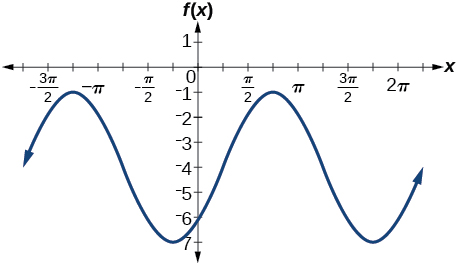6) $$f(x)=2\left (\cos\left ( x-\dfrac{4\pi }{3} \right )+1 \right )$$

7) $$f(x)=6\sin\left ( 3x-\dfrac{\pi }{6} \right )-1$$

amplitude: $$6$$; period: $$dfrac{2\pi }{3}$$; midline: $$y=-1$$; no asymptotes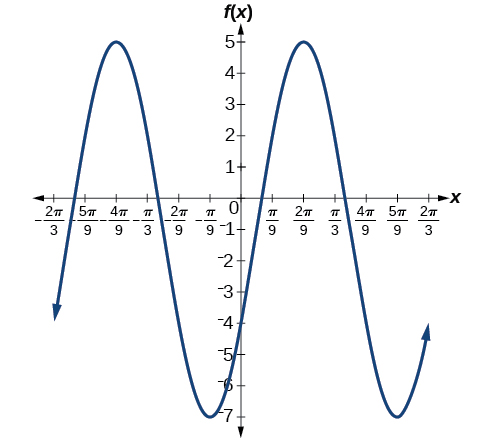8) $$f(x)=-100\sin(50x-20)$$

## 6.2: Graphs of the Other Trigonometric Functions

For the exercises 1-4, graph the functions for two periods and determine the amplitude or stretching factor, period, midline equation, and asymptotes.

1) $$f(x)=\tan x-4$$

stretching factor: none; period: $$\pi$$$;$midline: $$y=-4$$$;$asymptotes: $$x=\dfrac{\pi }{2}+\pi k$$, where $$k$$ is an integer2) $$f(x)=2\tan \left ( x-\dfrac{\pi }{6} \right )$$

3) $$f(x)=-3\tan (4x)-2$$

stretching factor: $$3$$; period: $$\dfrac{\pi }{4}$$; midline: $$y=-2$$; asymptotes: $$x=\dfrac{\pi }{8}+\dfrac{\pi }{4}k$$, where $$k$$ is an integer4) $$f(x)=0.2\cos(0.1x)+0.3$$

For the exercises 5-10, graph two full periods. Identify the period, the phase shift, the amplitude, and asymptotes.

5) $$f(x)=\dfrac{1}{3}\sec x$$

amplitude: none; period: $$2\pi$$; no phase shift; asymptotes: $$x=\dfrac{\pi }{2}k$$, where $$k$$ is an integer6) $$f(x)=3\cot x$$

7) $$f(x)=4\csc (5x)$$

amplitude: none; period: $$\dfrac{2\pi }{5}$$; no phase shift; asymptotes: $$x=\dfrac{\pi }{5}k$$, where $$k$$ is an integer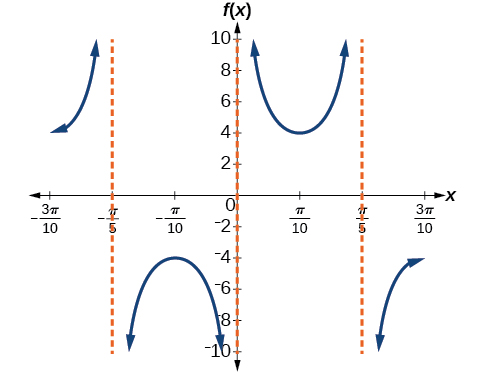8) $$f(x)=8\sec \left (\dfrac{1}{4}x \right )$$

9) $$f(x)=\dfrac{2}{3}\csc \left (\dfrac{1}{2}x \right )$$

amplitude: none; period: $$4\pi$$; no phase shift; asymptotes: $$x=2\pi k$$, where $$k$$ is an integer10) $$f(x)=-\csc (2x+\pi)$$

For the exercises 11-15, use this scenario: The population of a city has risen and fallen over a $$20$$-year interval. Its population may be modeled by the following function: $$y=12,000+8,000\sin(0.628x)$$, where the domain is the years since 1980 and the range is the population of the city.

11) What is the largest and smallest population the city may have?

largest: $$20,000$$; smallest: $$4,000$$

12) Graph the function on the domain of $$[0,40]$$.

13) What are the amplitude, period, and phase shift for the function?

amplitude: $$8,000$$; period: $$10$$; phase shift: $$0$$

14) Over this domain, when does the population reach $$18,000$$? $$13,000$$?

15) What is the predicted population in 2007? 2010?

In 2007, the predicted population is $$4,413$$. In 2010, the population will be $$11,924$$.

For the exercises 16a-16d, suppose a weight is attached to a spring and bobs up and down, exhibiting symmetry.

16) Suppose the graph of the displacement function is shown in the Figure below, where the values on the $$x$$-axis represent the time in seconds and the $$y$$-axis represents the displacement in inches.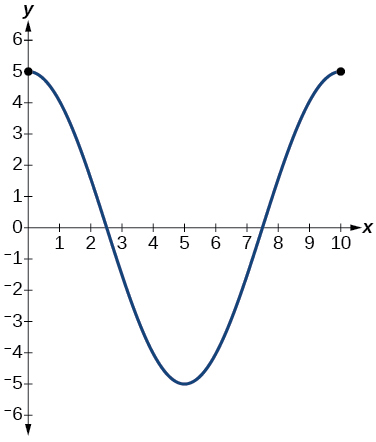1. Give the equation that models the vertical displacement of the weight on the spring.
2. At $$\text{time} = 0$$, what is the displacement of the weight?

$$5$$ in.

1. At what time does the displacement from the equilibrium point equal zero?
2. What is the time required for the weight to return to its initial height of $$5$$ inches? In other words, what is the period for the displacement function?

$$10$$ seconds

## 6.3: Inverse Trigonometric Functions

For the exercises 1-11, find the exact value without the aid of a calculator.

1) $$\sin ^{-1}(1)$$

2) $$\cos ^{-1}\left ( \dfrac{\sqrt{3}}{2} \right )$$

$$\dfrac{\pi }{6}$$

3) $$\tan ^{-1}(-1)$$

4) $$\cos ^{-1}\left ( \dfrac{1}{\sqrt{2}} \right )$$

$$\dfrac{\pi }{4}$$

5) $$\sin ^{-1}\left ( \dfrac{-\sqrt{3}}{2} \right )$$

6) $$\sin ^{-1}\left (\cos \left (\dfrac{\pi }{6} \right ) \right )$$

$$\dfrac{\pi }{3}$$

7) $$\cos ^{-1}\left (\tan \left (\dfrac{3\pi }{4} \right ) \right )$$

8) $$\sin \left (\sec^{-1} \left (\dfrac{3}{5} \right ) \right )$$

No solution

9) $$\cot \left (\sin^{-1} \left (\dfrac{3}{5} \right ) \right )$$

10) $$\tan \left (\cos^{-1} \left (\dfrac{5}{13} \right ) \right )$$

$$\dfrac{12}{5}$$

11) $$\sin \left (\cos^{-1} \left (\dfrac{x}{x+1} \right ) \right )$$

12) Graph $$f(x)=\cos x$$ and $$f(x)=\sec x$$ on the interval $$[0,2\pi )$$ and explain any observations.

The graphs are not symmetrical with respect to the line $$y=x$$.They are symmetrical with respect to the $$y$$-axis.13) Graph $$f(x)=\sin x$$ and $$f(x)=\csc x$$ and explain any observations.

14) Graph the function $$f(x)=\dfrac{x}{1}-\dfrac{x^3}{3!}+\dfrac{x^5}{5!}-\dfrac{x^7}{7!}$$ on the interval $$[-1,1]$$ and compare the graph to the graph of $$f(x)=\sin x$$ on the same interval. Describe any observations.

The graphs appear to be identical.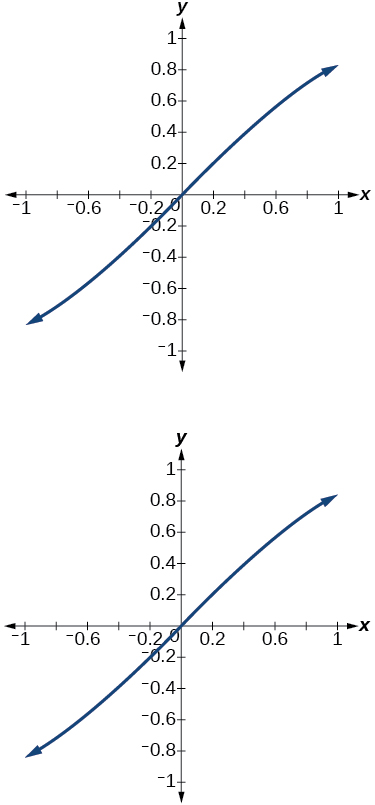## Practice Test

For the exercises 1-13, sketch the graph of each function for two full periods. Determine the amplitude, the period, and the equation for the midline.

1) $$f(x)=0.5\sin x$$

amplitude: $$0.5$$; period: $$2\pi$$$;$midline $$y=0$$